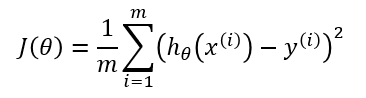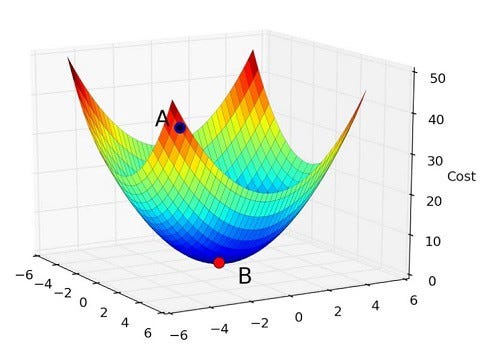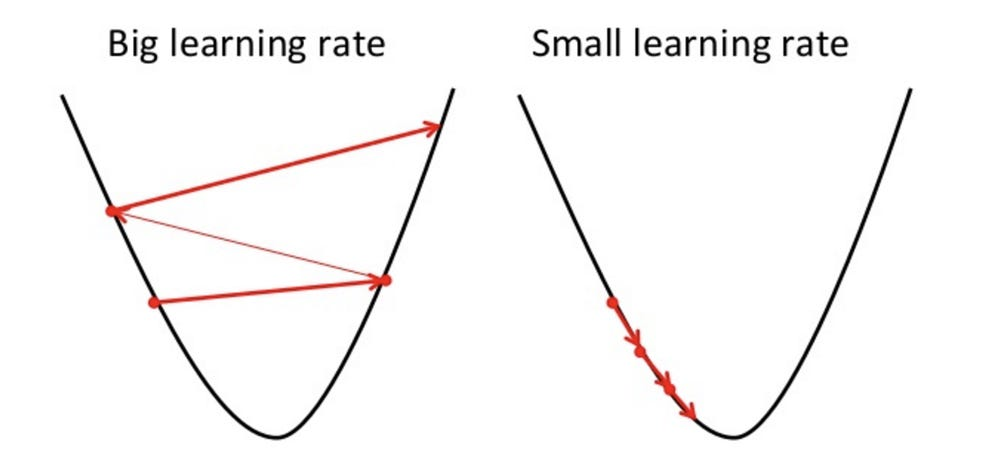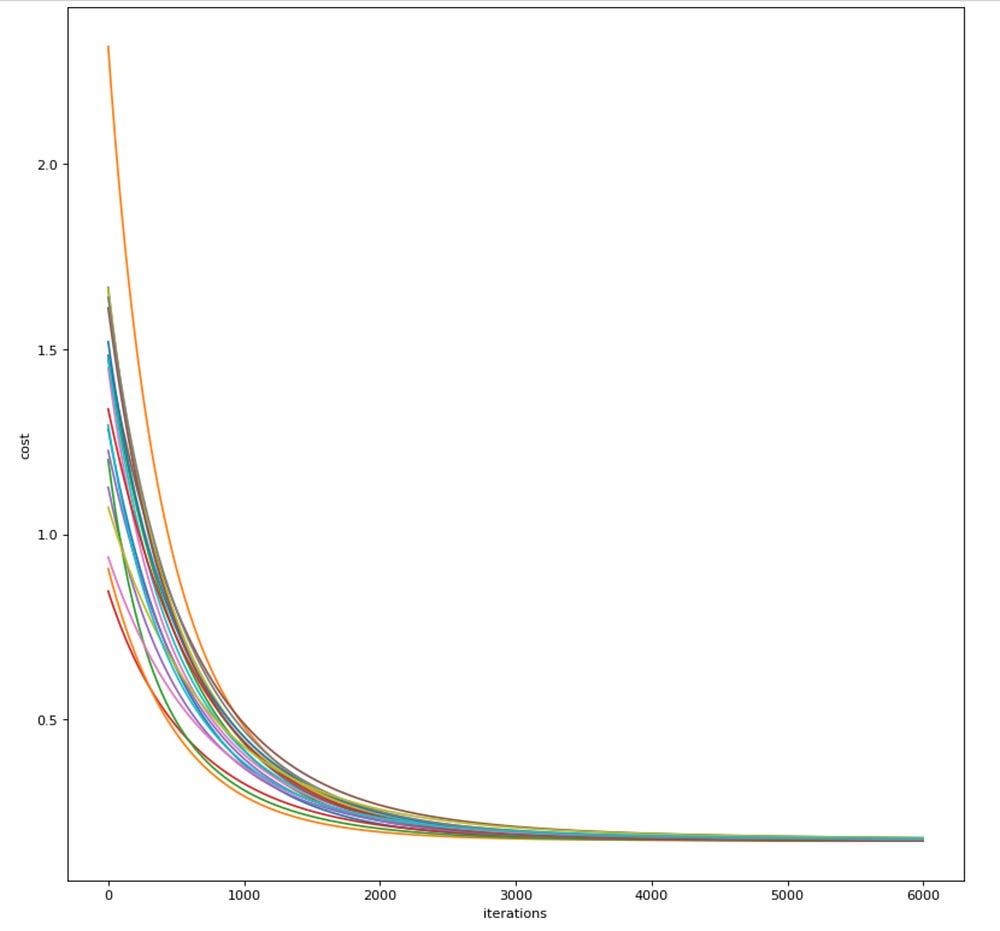Hitting the ground with Linear Regression

Source: Deep Learning on Medium

Statistically speaking, regression is a technique used to determine the statistical relationship between two or more variables where a change in a dependent variable is associated with, and depends on, a change in one or more independent variables.

So basically, if you were to remove out all the technical jargon, in layman’s terms, regression is a yet another name for curve-fitting. You will be given some input data, and our task is to figure out (a.k.a learn) a mathematical function which when given un-seen data predicts the output value which is as much close to the real output value as possible. By and large, this definition holds good for most, if not all, of the supervised machine learning techniques.

To solid-ify this concept, let us take an example. We will be using Airfoil Self-Noise Dataset from UCI ML repository. The dataset is as follows:

Important Note (Clarification): The input values for our problem are:

• Hertz — 1st input feature, denoted by x1
• Angle — 2nd input feature, denoted by x2
• Chord length — 3rd input feature, denoted by x3
• Velocity, and — 4th input feature, denoted by x4
• Thickness — 5th input feature, denoted by x5

The output value, which we are going to predict, is: Sound Pressure — denoted by y

By the definition of regression stated previously, we need to learn a function which is capable of calculating ‘Sound Pressure’ given Hertz, Angle, Chord length, Velocity and Thickness. Mathematically speaking, we need a function such as:

h(x) = θ0 + θ1×1 + θ2×2 + θ3×3 + θ4×4 + θ5×5

where, h(x) is the approximation for the actual output value ‘y’, and θ0, θ1, …, θ5 are the weights/parameters which we would like to determine by performing some magic!

Now, before diving into this problem, let us take a step back and visualise how would we solve the following equation (a rather simpler one):

y = m * x + c

Let us suppose, we have the following data at our disposal:

In the above picture, height is our x-variable (the input feature) and weight is our y-variable (the output feature.)

Remember, we need to do better than simply guessing ‘m’ and ‘c’ values — otherwise what’s the point of mathematics?

The Cost Function

In order to determine ‘m’ and ‘c’, let us simply set them to 0. Now, the equation is: y = 0

We, as humans, know that it is an absolute blunder to set ‘m’ and ‘c’ to zero for the above dataset. But how do we program the computer so that it knows how to evaluate whether a particular choice of parameters is poor or not. This is where cost function comes into picture. Cost function is a function which tells the computer whether a particular choice of parameters is optimal or not. Sometimes, we will refer to the computer as a model — which will be more apt in the field of machine learning.

There are several cost functions available, but one which is in our interests, and is also most widely used in regression based tasks is the Mean Squared Error (MSE) Cost Function, denoted by J(θ).Mean Squared Error (MSE) cost function (‘m’ is the total no:of examples and x(i), y(i) denote the i-th input example)

We use this cost function to measure how our model is performing against our chosen choice of parameters. As described previously, if we were to select ‘m’ and ‘c’ as zero, we will be getting a very high value of J(θ) — denoting that this selection is a poor choice.

Intuition behind MSE cost function

In MSE, the core operation being performed is the difference between the predicted output value, h(x) and the actual output value, y. This difference actually makes sense because if one wants to know how different one value is with respect to some other value, the most straightforward way to quantify such a difference is to subtract them. Furthermore, we square the difference because the predicted value might be greater than the actual value, or vice versa. It is crucial to remember that we would like to know the magnitude of difference, and not the direction of difference. The square function accomplishes this. However, there are other alternatives to get the magnitude of difference such as the modulus function (a.k.a absolute value). As a side note, traditionally, this difference is also called as an error.

It is not sufficient to simply calculate how different our model’s output value is from the actual output value for one example alone. The superscript ‘i’ denotes ‘i-th input example, and we perform a summation over all ‘m’ input examples and divide by the total number of examples to calculate the final difference between our model’s predictions and the actual output values.

Ok, so finally we are at a point to discuss how the learning in machine learning takes place. Restating again for the sake of clarity, the simplified equation which we are headed to solve is:

y = m * x + c

We figured out, with the help of a cost function, how to determine whether a particular choice of parameters (‘m’ and ‘c’ here) are good or bad. If the cost function outputs a zero value for our chosen ‘m’ and ‘c’ values, then Eureka! We have figured out the optimal parameters for our model. But in reality, this is far less likely to happen. Once our cost function outputs a positive value, we need to figure out a way to modify our parameters — read as tweaking the parameters — so that the new parameters yield a lower cost function value. If, god forbid, our cost function outputs a cost which is higher than the old value, then, clearly, something is wrong with the way we have updated our parameters.

What I have stated in the before paragraph is a rather extremely simplified example of gradient descent — the algorithm used for learning our model parameter values, ‘m’ and ‘c’.

Mathematically, gradient descent performs the minimisation of the cost function J(θ). Let us see how the plot of J(θ) with respect to the parameter values θ0 (or ‘c’ in our discussion) and θ1 (or ‘m’ in our discussion) actually looks like:x and y axis correspond to θ0 and θ1 respectively, whereas the z axis corresponds to the cost, J(θ)

Therefore, what we have done when we have chosen ‘m’ and ‘c’ values as zero, is that we have simply calculated the value of J(θ) at a particular point. Hence, our task now boils down to a standard optimisation problem in mathematics. Formally, we can describe gradient descent as follows: (Note: In the below figure ‘m’ is θ2 and ‘c’ is θ1)

To the uninitiated, at first sight, these mathematical terms might be daunting. You might question, are these the equations responsible for learning in machine learning? Yes they are!

There are two subtle things in the above equations which are responsible for learning: (While reading the below two points keep visualising about the 3D plot of J(θ) which we have plotted above)

• In which direction to descend? The slope of a curve (or a line for that matter) gives us a positive value when the curve is increasing and a negative value when the curve is decreasing. Furthermore, note that at a particular point, when a curve is increasing, then there will definitely be a minima towards its left, and vice-versa. Combining these two facts we get: “When the slope of a curve is positive, then move towards left and when slope is negative, move towards right — because that’s where minima lies”
• How much to descend in one step? This is our learning rate ⍺.Intuitively, to make the algorithm run faster we might want to choose as high a value as possible. While this logic seems to be right, there is a risk of over-shooting the minima and thereby making the algorithm slower to converge, or worse yet, diverging. Hence the step, in a single iteration, by which the gradient descent algorithm descends is the learning rate ⍺.

Note: Learning rate ⍺ is also called as a hyper-parameter. Hyper-parameter is a parameter whose value is set before the learning process begins. By contrast, the values of other parameters are derived via training. Moreover, there is no pre-defined method to choose the right set of hyper-parameters to a given model. The only way to set a hyper-parameter is by hit-and-trial. However, occasionally, one may come across hyper-parameter best-practises and start the learning process by choosing them. This is why the process of finding hyper-parameters is sometimes called hyper-parameter tuning.

Optional Information: If you are curious to know how the gradient descent works (or descends) when the learning rate, alpha, is varied:The curve is a 2D representation of the above cost function J(θ) and the direction and magnitude of the arrow represents the way learning rate varies

Linear Regression Model

We have talked about cost function and gradient descent. Let’s put them together in our linear regression model:

• Grab the input dataset. Figure out what are the features, and what is the output variable which we are going to predict.
• Initialise the weights of the features to zero
• Repeat the following two steps until convergence:
• (a) Calculate the cost of our model for the selected parameters
• (b) Update the parameters with gradient descent
• After convergence, you get the final parameters for your model which hopefully will give you the correct predictions for unseen data.

Convergence: What do we mean by convergence? Simply put, it is an instance where there is no point in making a parameter update using gradient descent because the cost function has remained constant for quite some time. This statement might seem like a soft constraint instead of a hard constraint, and I cannot agree more. Certain aspects of Machine learning is still more of an art than science, often filled with uncertainty. In fact, this is what makes the field all the more interesting.

Practically, one can plot the variation of the cost function at every iteration of the above loop, and if the implementation is correct, you will see a curve which is more or less similar to this:Graph containing several curves of the variation of the cost function with increasing number of iterations. Each curve represents a random initialisation of the parameter values

Evidently, we can see that after 4000 iterations, the cost function stagnates. This is how we determine the convergence.

Now, since we have understood what linear regression is, let us see it in practise by applying it to our Airfoil Self-Noise Dataset mentioned at the beginning of this article. We will be using python to implement the model.

h(x) = θ0 + θ1×1 + θ2×2 + θ3×3 + θ4×4 + θ5×5

From here on, you can follow along with me by referring to the IPython notebook present in my Github repository.

Here is the crux of the code:

Code Flow:

• Read and pre-process the dataset: The dataset is present in the repository in the form of a CSV file.We can read it via Pandas and visualise it by using matplotlib library. For more information, refer to the Github repository link where you will find additional code for all the utility functions. For illustration, here is the visualisation (scatter plot) of thickness (one of the input feature) and sound_pressure (the output variable.)

The primary advantage of visualisation is that, we can determine whether there are any redundant features by looking for correlation. Moreover, we can discard few data points which might seem like outliers, or check for class imbalance if we are performing classification. Other visualisations:

• Normalize the features: To ensure that one feature does not have more weightage than the other. For now, you can assume that this is the required step in every machine learning workflow. I will be writing another in-depth article on this later. Until then you can refer here.
• Split the dataset into training, dev, and test sets: Usually we do not use the entire dataset to perform our learning. Ideally, 80% of the data is dedicated to learning, remaining 20% is split equally between dev and train sets. A wonderful intuition is given here.
• Gradient descent: Run gradient descent to compute the optimal value of the parameters.
• Results: Finally, we calculate the RMSE (Root Mean Squared Error) of our model on train, dev and test sets to report the results. The intuition behind RMSE is same as that of MSE cost function. RMSE is one of the metrics which is used to determine how well a model is performing. An RMSE value of 0 means that the predicted result is exactly the same as the actual result, whereas a higher (non-zero) RMSE value means that the predicted result is different from that of the actual output value. For our model built for Airfoil Self-Noise dataset, the results are:

From the last example, we can see that for the datapoint:

• 6300 Hertz
• 5.3 Angle
• 0.2286 Chord length
• 39.6 Velocity
• 0.006 Thickness

We predicted 118.26 as the Sound Pressure. The actual value being 112.54. Pretty close! :D

Although we have achieved an RMSE accuracy of 4.87 on the test set, we can do much better. In this article we have tackled a supervised learning problem using linear regression — the easiest but not necessarily the effective technique for the problem at hand. Moreover, the model which have designed is a linear model:

h(x) = θ0 + θ1×1 + θ2×2 + θ3×3 + θ4×4 + θ5×5

In reality, many relationships are non-linear. Consider the problem of house price prediction with the area of the house for example. If the area of the house keeps on increasing, the price need not be increasing proportionately.

If you are already familiar with Machine Learning, you might be wondering what’s the point in implementing linear regression from scratch using Numpy. There are already several high-level libraries which do it for you. Such as, in Tensorflow:

``# Estimator using the default optimizer.estimator = LinearRegressor(feature_columns=[categorical_column_a,                     categorical_feature_a_x_categorical_feature_b])``

However, I adhere to the idea that if one wants to master a concept in computer science domain or otherwise, then he/she has to learn from the first principles.

Next week, we will have an article on neural networks — where we implement neural networks from scratch!